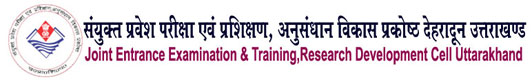Welcome to IRDT Uttarakhand E-Syllabus Web Portal01 CHEMICAL ENGGINEERING
 Duration : 03 Year Eligibility : 10th with Science & Mathematics Intake : 25/40/60(As approved by AICTE/State Govt.) Pattern of the Examination : Semester System Assessment Type : The assessment will we carry out through credit point system/Learning Based Out comes Theory & Practical Ratio : 50: 50 Industrial Training : Included after IInd Semester Ecology & Environment : As pe Government Directions a subject named Environmental Science & Energy Management has been included. Entrepreneurship Development : A subject on Entrepreneurship Development has been incorporated after IInd Semester. General Proficiency : A provision of 6 periods per week has been made. It Included of various cocurricular activities like games, hobby clubs, seminars, declamation contests, extension lectures, NCC, NSS, cultural activities and discipline etc.

### Syllabus

Paper Code :
992002
Paper Name :
APPLIED MATHEMATICS-II

#### Unit. I Co-ordinate Geometry

1.1 Equation of straight line in various standard forms (one point slope form, slope
intercept form, two point form, intercept form & normal form), inter section of
two straight lines, angle between two lines. Perpendicular distance formula.
1.2 General equation of a circle and its characteristics. To find the equation of a circle
given (i) Centre and radius (ii) Three points on it (iii) Co-ordinates of end points
of a diameter.
1.3 Equations of conics (ellipse, parabola and hyperbola), simple problems related
to engineering (standard forms only).

#### Unit – III Ordinary Differential

1.1- Definition, Order, Degree, Linear and Non-linear differential equatios.

1.2    Formation of differential equations ( upto second order).

1.3 Solution of first order differential equation-

(a) Variable Separable (b) Homogeneous (c) Reducible to Homogeneous (d) Linear differential

equation

(e) Bernoulli’s Equation (simple problem) (f) Exact differential Equation.

#### Unit – IV Statistics

1.1-Measures of Central Tendency: Mean, Median, Mode

1.2. Measures of Dispersion: Mean deviation, Standard deviation

1.3.  Co-efficient of rank correlation.

Website Developed By Softmaart